Share

# NCERT solutions for Class 7 Mathematics Textbook chapter 12 - Algebraic Expressions [Latest edition]

Course
Textbook page

#### Chapters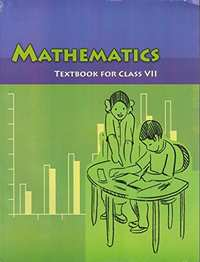## Chapter 12: Algebraic Expressions

Ex. 12.1Ex. 12.2Ex. 12.3Ex. 12.4

#### NCERT solutions for Class 7 Mathematics Textbook Chapter 12 Algebraic Expressions Exercise 12.1 [Pages 234 - 235]

Ex. 12.1 | Q 1.1 | Page 234

Get the algebraic expressions in the following cases using variables, constants and arithmetic operation

Subtraction of z from y

Ex. 12.1 | Q 1.2 | Page 234

Get the algebraic expressions in the following cases using variables, constants and arithmetic operation

One-half of the sum of numbers x and y.

Ex. 12.1 | Q 1.3 | Page 234

Get the algebraic expressions in the following cases using variables, constants and arithmetic operation

The number z multiplied by itself.

Ex. 12.1 | Q 1.4 | Page 234

Get the algebraic expressions in the following cases using variables, constants and arithmetic operation

One-fourth of the product of numbers p and q.

Ex. 12.1 | Q 1.5 | Page 234

Get the algebraic expressions in the following cases using variables, constants and arithmetic operation

Numbers x and y both squared and added

Ex. 12.1 | Q 1.6 | Page 234

Get the algebraic expressions in the following cases using variables, constants and arithmetic operation

Number 5 added to three times the product of number m and n.

Ex. 12.1 | Q 1.7 | Page 234

Get the algebraic expressions in the following cases using variables, constants and arithmetic operation

Product of numbers y and z subtracted from 10.

Ex. 12.1 | Q 1.8 | Page 234

Get the algebraic expressions in the following cases using variables, constants and arithmetic operation

Sum of numbers and b subtracted from their product

Ex. 12.1 | Q 2.1 | Page 234

Identify the terms and their factors in the following expressions

Show the terms and factors by tree diagrams.

a) x − 3

b)1 + x + x2

c) y − y3

d) 5xy2 +   7x2y

e) − ab + 2b2 − 3a2

Ex. 12.1 | Q 2.2 | Page 234

Identify terms and factors in the expressions given below:

a) − 4x + 5

b) − 4x + 5y

c) 5y + 3y2

d) xy + 2x2y2

e) pq + q

f) 1.2 ab − 2.4 b + 3.6 a

g) 3/4 x + 1/4

h) 0.1p2 + 0.2q2

Ex. 12.1 | Q 3 | Page 235

Identify the numerical coefficients of terms (other than constants) in the following expressions:

1) 5 − 3t2

2) 1 + t + t2 + t3

3) x + 2xy+ 3y

4) 100m + 1000n

5) − p2q2 + 7pq

6) 1.2a + 0.8b

7) 3.14 r2

8) 2 (l + b)

9) 0.1y + 0.01 y2

Ex. 12.1 | Q 4.1 | Page 235

Identify terms which contain x and give the coefficient of x.

1) y2x + y

2) 13y2− 8yx

3) x + y + 2

4) 5 + z + zx

5) 1 + x+ xy

6) 12xy2 + 25

7) 7x + xy2

Ex. 12.1 | Q 4.2 | Page 235

Identify terms which contain y2 and give the coefficient of y2.

1) 8 - xy2

2) 5y2 + 7x

3) 2x2y - 15xy2 + 7y2

Ex. 12.1 | Q 5.01 | Page 235

Classify into monomials, binomials and trinomials

4y − 7z

Ex. 12.1 | Q 5.02 | Page 235

Classify into monomials, binomials and trinomials

y2

Ex. 12.1 | Q 5.03 | Page 235

Classify into monomials, binomials and trinomials.

x + y − xy

Ex. 12.1 | Q 5.04 | Page 235

Classify into monomials, binomials and trinomials

100

Ex. 12.1 | Q 5.05 | Page 235

Classify into monomials, binomials and trinomials

ab − a − b

Ex. 12.1 | Q 5.06 | Page 235

Classify into monomials, binomials and trinomials

5 − 3t

Ex. 12.1 | Q 5.07 | Page 235

Classify into monomials, binomials and trinomials

4p2q − 4pq2

Ex. 12.1 | Q 5.08 | Page 235

Classify into monomials, binomials and trinomials

7mn

Ex. 12.1 | Q 5.09 | Page 235

Classify into monomials, binomials and trinomials

z2 − 3z + 8

Ex. 12.1 | Q 5.1 | Page 235

Classify into monomials, binomials and trinomials

a2 + b2

Ex. 12.1 | Q 5.11 | Page 235

Classify into monomials, binomials and trinomials

z2 + z

Ex. 12.1 | Q 5.12 | Page 235

Classify into monomials, binomials and trinomials

1 + x + x2

Ex. 12.1 | Q 6.1 | Page 235

State whether a given pair of terms is of like or unlike term

1, 100

Ex. 12.1 | Q 6.2 | Page 235

State whether a given pair of terms is of like or unlike term

-7x, 5/2 x

Ex. 12.1 | Q 6.3 | Page 235

State whether a given pair of terms is of like or unlike terms.

− 29x, − 29y

Ex. 12.1 | Q 6.4 | Page 235

State whether a given pair of terms is of like or unlike terms.

14xy, 42yx

Ex. 12.1 | Q 6.5 | Page 235

State whether a given pair of terms is of like or unlike terms.

4m2p, 4mp2

Ex. 12.1 | Q 6.6 | Page 235

State whether a given pair of terms is of like or unlike terms.

12xz, 12 x2z2

Ex. 12.1 | Q 7.1 | Page 235

Identify like terms in the following:

−xy2, − 4yx2, 8x2, 2xy2, 7y, − 11x2, − 100x, −11yx, 20x2y, −6x2, y, 2xy,3x

Ex. 12.1 | Q 7.2 | Page 235

Identify like terms in the following:

10pq, 7p, 8q, − p2q2, − 7qp, − 100q, − 23, 12q2p2, − 5p2, 41, 2405p, 78qp, 13p2q, qp2, 701p2

#### NCERT solutions for Class 7 Mathematics Textbook Chapter 12 Algebraic Expressions Exercise 12.2 [Pages 239 - 240]

Ex. 12.2 | Q 1.1 | Page 239

Simplify combining like terms: 21b − 32 + 7b − 20b

Ex. 12.2 | Q 1.2 | Page 239

Simplify combining like terms: - z2 + 13z2 − 5z + 7z3 − 15z

Ex. 12.2 | Q 1.3 | Page 239

Simplify combining like terms: p − (p − q) − q − (− p)

Ex. 12.2 | Q 1.4 | Page 239

Simplify combining like terms: 3a - 2b - ab - (a - b + ab) + 3ab + b - a

Ex. 12.2 | Q 1.5 | Page 239

Simplify combining like terms: 5x2y − 5x2 + 3y x2 − 3y2 + x2 − y2 + 8xy2 −3y2

Ex. 12.2 | Q 1.6 | Page 239

Simplify combining like terms: (3y2 + 5y - 4) - (8y - y2 - 4)

Ex. 12.2 | Q 2.01 | Page 239

Add: 3mn, − 5mn, 8mn, −4mn

Ex. 12.2 | Q 2.02 | Page 239

Add: t - 8tz, 3tz - z, z - t

Ex. 12.2 | Q 2.03 | Page 239

Add: -7mn + 5, 12mn + 2, 9mn - 8, -2mn - 3

Ex. 12.2 | Q 2.04 | Page 239

Add: a + b - 3, b - a + 3, a - b + 3

Ex. 12.2 | Q 2.05 | Page 239

Add: 14x + 10y - 12xy - 13, 18 - 7x - 10y + 8xy, 4xy

Ex. 12.2 | Q 2.06 | Page 239

Add: 5m - 7n, 3n - 4m + 2, 2m - 3mn - 5

Ex. 12.2 | Q 2.07 | Page 239

Add: 4x2y, - 3xy2, - 5xy2, 5x2y

Ex. 12.2 | Q 2.08 | Page 239

Add: 3p2q2 - 4pq + 5, - 10p2q2, 15 + 9pq + 7p2q2

Ex. 12.2 | Q 2.09 | Page 239

Add:ab - 4a, 4b - ab, 4a - 4b

Ex. 12.2 | Q 2.1 | Page 239

Add: x2 - y2 - 1 , y2 - 1 - x2, 1- x2 - y2

Ex. 12.2 | Q 3.1 | Page 240

Subtract: - 5y2 from y2

Ex. 12.2 | Q 3.2 | Page 240

Subtract: 6xy from − 12xy

Ex. 12.2 | Q 3.3 | Page 240

Subtract: (a - b) from (a + b)

Ex. 12.2 | Q 3.4 | Page 240

Subtract: a (b - 5) from b (5 - a)

Ex. 12.2 | Q 3.5 | Page 240

Subtract: -m2 + 5mn from 4m2 - 3mn + 8

Ex. 12.2 | Q 3.6 | Page 240

Subtract: -x2 + 10x - 5 from 5x - 10

Ex. 12.2 | Q 3.7 | Page 240

Subtract: 5a2 - 7ab + 5b2 from 3ab - 2a2 -2b2

Ex. 12.2 | Q 3.8 | Page 240

Subtract: 4pq - 5q2 - 3p2 from 5p2 + 3q2 - pq

Ex. 12.2 | Q 4.1 | Page 240

What should be added to x2 + xy + y2 to obtain 2x2 + 3xy?

Ex. 12.2 | Q 4.2 | Page 240

What should be subtracted from 2a + 8b + 10 to get - 3a + 7b + 16?

Ex. 12.2 | Q 5 | Page 240

What should be taken away from 3x2 - 4y2 + 5xy + 20 to obtain - x2 - y2 + 6xy + 20?

Ex. 12.2 | Q 6.1 | Page 240

From the sum of 3x - y + 11 and - y - 11, subtract 3x - y - 11.

Ex. 12.2 | Q 6.2 | Page 240

From the sum of 4 + 3x and 5 - 4x + 2x2, subtract the sum of 3x2 - 5x and -x2 + 2x + 5.

#### NCERT solutions for Class 7 Mathematics Textbook Chapter 12 Algebraic Expressions Exercise 12.3 [Page 242]

Ex. 12.3 | Q 1.1 | Page 242

If m = 2, find the value of m − 2

Ex. 12.3 | Q 1.2 | Page 242

If m = 2, find the value of 3m − 5

Ex. 12.3 | Q 1.3 | Page 242

If m = 2, find the value of 9 - 5m

Ex. 12.3 | Q 1.4 | Page 242

If m = 2, find the value of: 3m2 − 2m − 7

Ex. 12.3 | Q 1.5 | Page 242

If m = 2, find the value of: "5m"/2 - 4

Ex. 12.3 | Q 2.1 | Page 242

If p = −2, find the value of: 4p + 7

Ex. 12.3 | Q 2.2 | Page 242

If p = -2, find the value of −3p2 + 4p + 7

Ex. 12.3 | Q 2.3 | Page 242

If p = -2, find the value of -2p3 - 3p2 + 4p + 7

Ex. 12.3 | Q 3.1 | Page 242

Find the value of the following expressions, when x = − 1:

2x - 7

Ex. 12.3 | Q 3.2 | Page 242

Find the value of the following expressions, when x = - 1:

-x + 2

Ex. 12.3 | Q 3.3 | Page 242

Find the value of the following expressions, when x = -1:

x2 + 2x + 1

Ex. 12.3 | Q 3.4 | Page 242

Find the value of the following expressions, when x = − 1:

2x2 − x − 2

Ex. 12.3 | Q 4.1 | Page 242

If a = 2, b = − 2, find the value of a2 + b2

Ex. 12.3 | Q 4.2 | Page 242

If a = 2, b = − 2, find the value of a2 + ab + b2

Ex. 12.3 | Q 4.3 | Page 242

If a = 2, b = − 2, find the value of a2 − b2

Ex. 12.3 | Q 5.1 | Page 242

When a = 0, b = − 1, find the value of the given expressions 2a + 2b

Ex. 12.3 | Q 5.2 | Page 242

When a = 0, b = − 1, find the value of the given expressions 2a2 + b2 + 1

Ex. 12.3 | Q 5.3 | Page 242

When a = 0, b = − 1, find the value of the given expressions 2a2 b + 2ab2 + ab

Ex. 12.3 | Q 5.4 | Page 242

When a = 0, b = − 1, find the value of the given expressions a2 + ab + 2

Ex. 12.3 | Q 6.1 | Page 242

Simplify the expressions and find the value if x is equal to 2:

x + 7 + 4 (x - 5)

Ex. 12.3 | Q 6.2 | Page 242

Simplify the expressions and find the value if x is equal to 2 :

3 (x + 2) + 5x - 7

Ex. 12.3 | Q 6.3 | Page 242

Simplify the expressions and find the value if x is equal to 2:

6x + 5 (x − 2)

Ex. 12.3 | Q 6.4 | Page 242

Simplify the expressions and find the value if x is equal to 2:

4 (2x - 1) + 3+ 11

Ex. 12.3 | Q 7.1 | Page 242

Simplify these expressions and find their values if x = 3, a = − 1, b = − 2.

3x - 5 - x + 9

Ex. 12.3 | Q 7.2 | Page 242

Simplify these expressions and find their values if x = 3, a = − 1, b = − 2

2 - 8x + 4x + 4

Ex. 12.3 | Q 7.3 | Page 242

Simplify these expressions and find their values if x = 3, a = − 1, b = − 2

3a + 5 - 8a + 1

Ex. 12.3 | Q 7.4 | Page 242

Simplify these expressions and find their values if x = 3, a = − 1, b = − 2

10 - 3b - 4 - 5b

Ex. 12.3 | Q 7.5 | Page 242

Simplify these expressions and find their values if x = 3, a = − 1, b = − 2.

2a - 2b - 4 - 5 + a

Ex. 12.3 | Q 8.1 | Page 242

if z = 10, find the value of z3 − 3 (z − 10).

Ex. 12.3 | Q 8.2 | Page 242

If p = − 10, find the value of p2 − 2p − 100

Ex. 12.3 | Q 9 | Page 242

What should be the value of a if the value of 2x2 + x − a equals to 5, when x = 0?

Ex. 12.3 | Q 10 | Page 242

Simplify the expression and find its value when a = 5 and b = −3.

2 (a2 + ab) + 3 − ab

#### NCERT solutions for Class 7 Mathematics Textbook Chapter 12 Algebraic Expressions Exercise 12.4 [Pages 246 - 247]

Ex. 12.4 | Q 1.1 | Page 246

Observe the patterns of digits made from line segments of equal length. You will find such segmented digits on the display of electronic watches or calculators.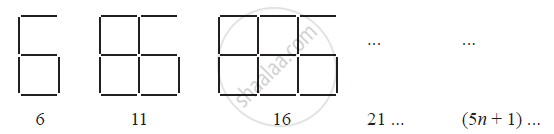If the number of digits formed is taken to be n, the number of segments required to
form n digits is given by the algebraic expression appearing on the right of each pattern.

How many segments are required to form 5, 10, 100 digits of the kind −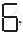Ex. 12.4 | Q 1.2 | Page 246

Observe the patterns of digits made from line segments of equal length. You will find such segmented digits on the display of electronic watches or calculators.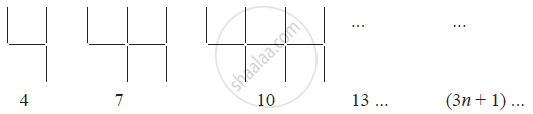If the number of digits formed is taken to be n, the number of segments required to form n digits is given by the algebraic expression appearing on the right of each pattern.

How many segments are required to form 5, 10, 100 digits of the kind −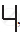Ex. 12.4 | Q 1.3 | Page 246

Observe the patterns of digits made from line segments of equal length. You will find such segmented digits on the display of electronic watches or calculators.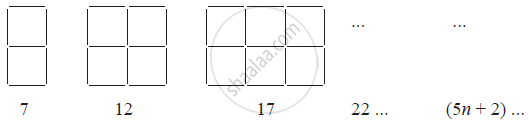If the number of digits formed is taken to be n, the number of segments required to form n digits is given by the algebraic expression appearing on the right of each pattern.

How many segments are required to form 5, 10, 100 digits of the kind −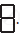Ex. 12.4 | Q 2 | Page 247

Use the given algebraic expression to complete the table of number patterns.

 S. No Expression Terms 1st 2nd 3rd 4th 5th ... 10th ... 100th ... 1 2n - 1 1 3 5 7 9 - 19 - - - 2 3n + 2 2 5 8 11 - - - - - - 3 4n + 1 5 9 13 17 - - - - - - 4 7n + 20 27 34 41 48 - - - - - - 5 n2 + 1 2 5 10 17 - - - - 10001 -

## Chapter 12: Algebraic Expressions

Ex. 12.1Ex. 12.2Ex. 12.3Ex. 12.4## NCERT solutions for Class 7 Mathematics Textbook chapter 12 - Algebraic Expressions

NCERT solutions for Class 7 Mathematics Textbook chapter 12 (Algebraic Expressions) include all questions with solution and detail explanation. This will clear students doubts about any question and improve application skills while preparing for board exams. The detailed, step-by-step solutions will help you understand the concepts better and clear your confusions, if any. Shaalaa.com has the CBSE Class 7 Mathematics Textbook solutions in a manner that help students grasp basic concepts better and faster.

Further, we at Shaalaa.com provide such solutions so that students can prepare for written exams. NCERT textbook solutions can be a core help for self-study and acts as a perfect self-help guidance for students.

Concepts covered in Class 7 Mathematics Textbook chapter 12 Algebraic Expressions are Concept of Expressions Formed, Terms of an Expression, Like and Unlike Terms, Monomials, Binomials, Trinomials and Polynomials, Addition and Subtraction of Algebraic Expressions, Finding the Value of an Expression, Using Algebraic Expressions – Formulas and Rules.

Using NCERT Class 7 solutions Algebraic Expressions exercise by students are an easy way to prepare for the exams, as they involve solutions arranged chapter-wise also page wise. The questions involved in NCERT Solutions are important questions that can be asked in the final exam. Maximum students of CBSE Class 7 prefer NCERT Textbook Solutions to score more in exam.

Get the free view of chapter 12 Algebraic Expressions Class 7 extra questions for Class 7 Mathematics Textbook and can use Shaalaa.com to keep it handy for your exam preparation

S